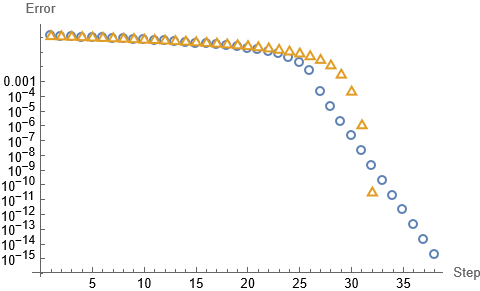# Newton's Method: A Control Theoretic Perspective

Consider the problem of finding an $$x_* \in \mathbb{R}$$ so that, $f(x_*) = 0,$ for some given continuously differentiable function $$f: \mathbb{R} \to \mathbb{R}.$$ Also assume $$f'(x_*) \neq 0.$$ One of the most powerful, yet simple, methods to find this solution is Newton’s Method. Newton’s method involves computing iterates $$x_k$$ starting at some initial value $$x_0$$ near $$x_*$$ according to the formula, $x[k+1] = x[k] - \frac{f(x[k])}{f'(x[k])}.$ Why this formula? This post takes a different perspective than the literature, and we will view the problem of solving $$f(x_*) = 0$$ as a stabilization problem for discrete-time control systems.

Consider the discrete-time control system, $x[k+1] = x[k] + u[k],$ with inputs $$u[k] \in \mathbb{R}^n.$$ From a control theory perspective, this is just an integrator. It is an “update” equation from a numerical algorithms perspective. Define an output $z[k] = f(x[k]),$ and see that it observes the discrete-time law, $z[k+1] = f(x[k] + u[k])$ Write a second-order Taylor series expansion for $$f$$ about the current observation $$z[k] = f(x[k])$$ to get, $z[k+1] = z[k] + f'(x[k]) u[k] + \mathscr{O}(u[k]^2).$ Write a new input $$v[k]$$ in terms of $$u[k]$$ by the formula, $v[k] = f'(x[k]) u[k].$ The recurrence relation for $$z[k]$$ then takes the form, $z[k+1] = z[k] + v[k] + \mathscr{O}(v[k]^2).$ Introduce a proportional feedback controller, $v[k] = K z[k],$ resulting in the final dynamics, $z[k+1] = (1 + K) z[k] + \mathscr{O}(z[k]^2).$ Discrete-time system theory tells us that we have local exponential stability if the Jacobian of the right-hand side about $$0$$ has eigenvalues in the unit disc; this occurs when $$K \in (-2, 0)$$ Such a choice gives local exponential stability of $$x[k]$$ to $$x_*$$ since $$f$$ is locally linear. Great, so what is the final stepping equation we have concocted? Working backwards, i.e. writing $$u[k]$$ in terms of $$x[k]$$ and $$K,$$ we can solve for the final stepping equation, $x[k+1] = x[k] + K \frac{f(x[k])}{ f'(x[k]) }.$ Notice that, when $$K = -1,$$ we recover the standard Newton’s method and the linearization of the discrete-time system achieves the fastest possible theoretical convergence: finite-time convergence. This is where the state $$x[k]$$ converges in a finite number of iterations. Although this is the best possible theoretical design, such implementations are generally avoided in control applications due to their lack of robustness to noise. Returning back to our choices for $$K$$, note that for $$K \in (-2, 0) \setminus \{-1\}$$ we recover a subclass of the modified Newton’s method. Thus, we have shown that Newton’s method and a class of variants achieve local exponential convergence to the root $$x_*.$$Figure 1: Convergence of the error $$x[k] - x_*$$ in the log-scale of Newton’s method (Yellow, Triangle) and a modified Newton’s method with gain $$K = -1.1$$ (Blue, Circle).

In Figure 1, the error in $$x[k]$$ for solving the problem of finding a root to, $f(x) = (x - 5)^{50} (x - \pi),$ are shown with the initial condition $$x = 2.$$ Notice that the convergence of Newton’s method and the modified approach with $$K=-1.1$$ have effectively the same convergence until the error gets close to zero. At near zero error, Newton’s method accelerates to a solution near machine precision at faster-than exponential convergence, while the modified method speeds up but retains an exponential convergence.

Readers should compare this result to the more standard result that, under slightly stricter assumptions, Newton’s method has the property that, $0 < \lim_{k \to \infty} \frac{\|x[k+1] - x_*\|}{\|x[{k}] - x_*\|^2} < \infty.$ This is known as quadratic convergence in the numerical computation literature and is a stronger notion of convergence. Exponential stability of a discrete-time control system falls under the category of linear convergence in the numerical computation literature: its convergence is linear since the error $$x[k] - x_*$$ falls by a constant multiple of the error. Quadratic convergence of Newton’s method relies on a continuous second derivative and an order one root, while the linear convergence established here only relies on twice differentiability and a continuous first derivative. Although a weaker result, analyzing numerical algorithms in a control theoretic way lends these techniques to interconnection with other numerical algorithms. The convergence of these interconnected algorithms is then supported by control theory without further technical analysis.